# Accuracy, uncertainty and linear alteration of geographic data (1)

It is not easy, in geomatics, to get a good grasp of the geodesy notions that underlie the manipulation of geographic data. Unfortunately, many users do not have a sound knowledge of these concepts. I have to admit, that this knowledge can become superfluous if, for example, all your data is in the same coordinate system.

Each of these terms implies a different notion. And the problem becomes even more complicated when we say that the gap we are talking about is due to the combination of these three concepts (and others that we have not yet named).

We can, already, say that to determine this gap we must firstly state that its determination will be useful to measure it. Yes, our problem is more complicated than others, but, on the other side, it means that we have a whole range of possibilities to express the location of an entity in the space. The two best-known methodologies is the use of latitudes/longitudes (geographic coordinate system) or metric XY coordinates (metric coordinate system).

A significant contribution to the development of GIS tools has been the introduction of the on the fly projection: you can use the data in different systems while displaying and processing them into your workspace, the software that performs the necessary coordinates transformations. The issue with these black boxes is that the user has been relieved of the need to know how these transformations are done as well as what they might imply as problems for the quality of the data.

### A few “definitions”

The main characteristics of measuring instruments (or metrological properties of measuring devices) are defined within the framework of the International Vocabulary of Metrology and include, among others:

•  the resolution
•  the accuracy
•  the exactitude

Note that the term “precision” is not used and is considered as a language error in metrology. If you search the International Vocabulary of Metrology   for the term “Precision” you will find nothing. The ” precision “ does not exist in metrology!

On the other hand   the precision   is very much used in computer science. Any measurement made using a device gives you a result that is never the true value of the quantity being measured. Even in the absence of precision given explicitly on a result, the simple numerical value implies a precision by the only number of significant digits indicated.  The figures given in the result are jus those that make sense, which means that the next digit would not make sense in the context of the measurement. This is why we depict 6 decimal places in our geographic position values, for example on a layer in Lambert 93, would mean that the position is accurate to the micrometer!

The resolution   does not fall within the scope of this article. The resolution of an apparatus is the smallest variation of the measured quantity that causes a perceivable variation of the instrument reading.

The accuracy is also linked to the measuring device. The higher the coincidence between the “true value” and the instrument reading, the higher the accuracy of the measuring device. It has to be highlighted that the accuracy is not expressed by a numerical value. It is a qualitative assessment of the results. Apart from the operating conditions, the accuracy of a device is essentially related to its accuracy and fidelity. A device is accurate if it is both accurate and faithful.

We can represent symbolically   fidelity ,   exactitude and accuracy   as follows:(Fidelite=Fidelity, Justesse=Exactitude, Exactitude=Accuracy)

In the first option, the measurements are close to each other (good fidelity) but outside the probability zone of the true value (bad accuracy).

In the second option, the measurements in the area where the true value is located are the opposite, and the “centre of gravity” of the points is in the centre of the red zone (good accuracy) but although good, the measurements are scattered ( bad fidelity).

Finally, the last option depicts correct measures (in the true value area) and faithfulness (close to each other). This is the case of a good measuring device, where a correction is, a priori, not necessary and the measurements made with the device are accurate.

Our interest resides in the accuracy of the location of our objects in our information system.

To understand it, we will track the acquisition of data, from a GPS to its inclusion in an information system.

### Astronomical coordinates

We will begin with astronomical latitude and longitude, because even though they will not be useful to us in the context of a GIS, they allow introducing some aspects that we will be using in the other systems.

The astronomical latitude ( Φ ) of a point is given by the angle formed between the direction of the vertical at this point, and the equatorial plane.

Its astronomical (or true) meridian is defined as the plane passing through the vertical and the Earth rotation axis: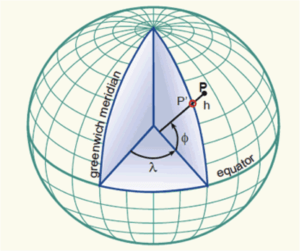Conventionally, the prime meridian is   the astronomical meridian that passes through the telescope of the former Greenwich Observatory. Therefore the longitude of a point ( λ ) is the angle between two planes, one of which is the local meridian (or the meridian plane) and the Greenwich meridian.

As you could see in the previous figure, the vertical of the point on the Earth’s surface does not necessarily go through the centre of the Earth (intersection of the equatorial and meridian planes and the axis of rotation of the Earth).

It would always pass through the centre if the Earth were a perfect sphere, but as the Earth’s surface is a geoids (dented balloon) you can have multiple points with exactly parallel verticals and so … with the same astronomical latitude!

The following figure shows four places with the same astronomical latitude: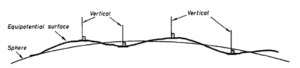Then what is the point? Well, as its name suggests, it is useful in astronomical terms. The astronomical latitude and longitude is used to position the stars relative to the place of observation. On the other hand they are of no use to locate our entities in a GIS.

We will use the first figure to understand another coordinate system.

### Geocentric coordinates (GPS)

If we take the same point on the earth’s surface and use an imaginary line connecting this point to the centre of the Earth, we can calculate the two angles formed (latitude and longitude). On the other hand, this line will not be perpendicular to the surface where the point is located.

Here is a diagram with the two types of positioning we have just discussed ( Φ : astronomical latitude and Φ ‘: geocentric latitude)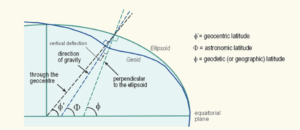Later in this article, we will discuss the third type of latitude (geodesic latitude).

The GPS provides geocentric coordinates.

What to remember? First of all, contrary to common belief, the coordinates are constituted by three values ​​and not two: latitude, longitude and height. The geocentric latitude and longitude defines the dotted line ( Φ ‘) of the figure. The height (in meters from the centre of the Earth) defines the position of the point on this line.

The second thing to remember is that the whole system is based on the position of the centre of the Earth (intersection of the rotation axis and the equatorial plane). As the earth is not a perfect sphere, the definition of this centre has to be calculated. Inevitably, there has been a plethora of different calculations made by scientists from different countries.

So the GPS system has chosen a centre of the Earth. The WGS84 has been chosen and  adopted as the standard system for the so-called   global systems . Global systems, as opposed to local systems, can be used anywhere on Earth.

This coordinate system is perfect when defining the position of a point, but presents a problem when used in an information system: it is not possible to accurately calculate a distance between two places on the Earth’s surface, because it would be necessary to know all the hollows and bumps of the geoids between these two points, or at least to know a priori all the heights (third parameter)of the points that can be part of the information system.

Since this is very complicated, we have adopted a simpler solution: replace the real surface of the geoids by a smooth and regular surface: an ellipsoid.

### Geodetic coordinates

An ellipsoid is as follows:The geodesic position of a point on the Earth surface is defined by the ellipsoidal coordinates of the projection of that point on the surface of a reference ellipsoid, along the normal to that ellipsoid. The geodesic latitude ( Φ ) is defined as the inclination of the equatorial plane normal to ellipsoidal, and the geodesic meridian as the plane passing through the normal axis and the minor axis of the reference ellipsoid. Finally, the geodesic longitude ( λ ) of a point is the angle between its meridian plane and the reference meridian.Unlike the astronomical coordinates of the points on the Earth’s surface, no point of projection on the reference ellipsoid can have identical geodesic coordinates. Therefore, the same applies for the geodesic coordinates of two points on the Earth surface, unless they are placed on the same normal as the ellipsoid. In addition, as with the plane and surface of the sphere, one can develop formulas and calculate the distance (ellipsoidal) and azimuth between two points whose geodesic coordinates (ellipsoid) are known. The reason for using a rotating ellipsoid rather than a plane, sphere or cube as a geodesic reference surface is a matter of convenience (and not theoretical rigour), since the ellipsoid of rotation is the regular geometrical form best fitted to the real shape of the Earth that helps us solve the two basic geodesic problems. In other words, one can develop formulas for calculating the distance and the azimuth between two points of known coordinates and the coordinates of a second point whose azimuth and the distance of a first known point are given. The size (that is, the major axis,  a , and flattening,   f ) of the reference ellipsoid can be chosen arbitrarily, but the positioning of the ellipsoid relative to the solid Earth is of crucial importance. In classical geodetic practice, this is done by arbitrarily adopting values ​​of geodesic latitude, longitude, and height above the ellipsoid for a station of origin (the Paris Pantheon Cross in the case of the New French Triangulation, NTF), and using mathematical formulas that maintain the parallelism between the minor axis of the ellipsoid and the mean axis of rotation of the Earth.

It’s complicated, but let’s get to the basics, because geodesic coordinates are the coordinates used in GIS.

First of all, each geodesic system (and there are plenty) is characterized by the choice of a centre of the Earth, then by a model of ellipsoid.

Take three common systems used for France: the WGS84, the RGF93 and the NTF. The first one is used for UTM a map, the second one is used by Lambert 93 coverage, the third by Lambert NTF projection on IGN (National French Institute) maps.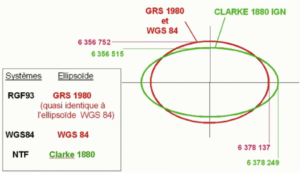As you can see, the ellipsoids are different. The world according to NTF is more flattened. In the figure we do cannot appreciate the second difference, which is the position of the centre of the Earth.

The two systems locate the centre of the Earth in two different places: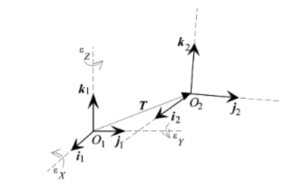They are not “ too “ far away  but still they are separated by 168m in X, 60m in Y and 320m in Z. This difference makes that the same position on the Earth surface (same Latitude and Longitude) be separated by a distance of up to a hundred meters .

But now, we have another problem. Using an ellipsoid means that we will only have two parameters to define the position of a point: latitude and longitude. The third parameter, the Height (that we used with the geocentric coordinates) disappears because any position is brought back to the surface of the ellipsoid, the point P of the following figure.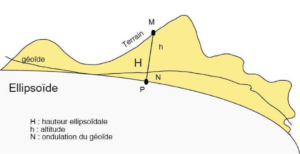In a geodesic coordinate system, the position of the point M becomes the point P. We move from a three-dimensional system (XYZ) to a two-dimensional system (XY). To work in 3D it is necessary to give a third element that can be either the ellipsoidal height or the altitude. Altitudes are measured relative to the surface of the geoids. In order to know them, you have to measure them on the spot. On the other hand a GPS provides the ellipsoidal height H, because it can calculate the difference between the Z measured in geocentric coordinates and the terrestrial surface formula adopted with the ellipsoid.

In the previous figure it should be understood that H = h + N. But if you work with a GPS only H can be measured.

In the following article we will focus on the factors that affect the quality of data in a geographic information system.